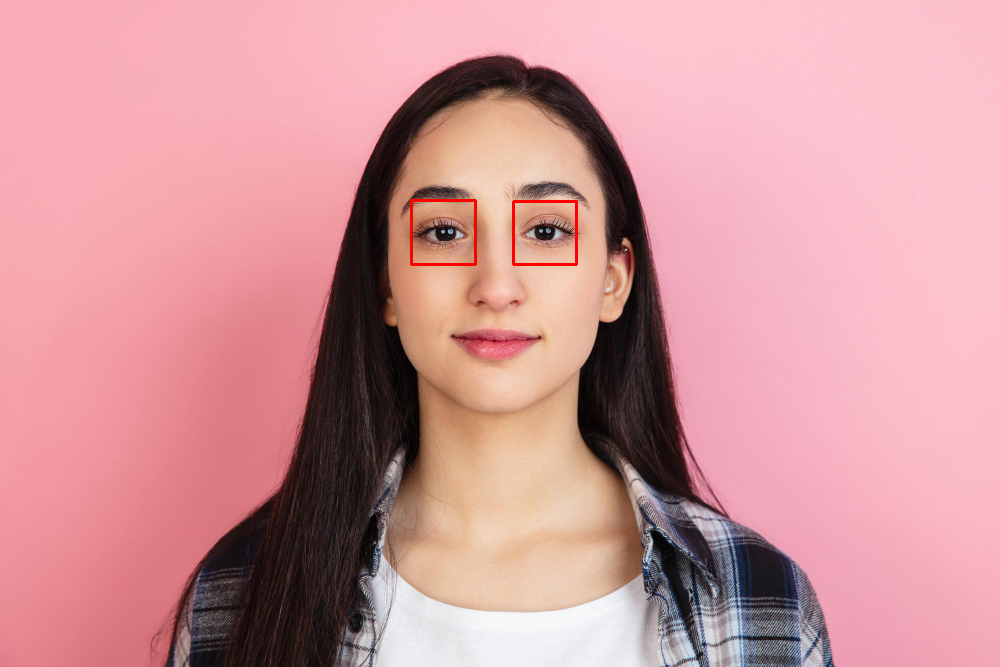• # Eye Detection with OpenCV

• Eye detection is a popular and useful operation in OpenCV.

• It can be used in a variety of applications including face recognition systems and driver fatigue detection.

• This process use Haar cascades machine learning technique.

• We can also use self trained models in this process.

In this tutorial, we’ll learn about eye detection with OpenCV.

Input:Code:

``````import cv2

gray = cv2.cvtColor(input_image, cv2.COLOR_BGR2GRAY)

for (x, y, w, h) in eye:
img = cv2.rectangle(input_image, (x, y), (x + w, y + h), (0, 0, 255), 2)

cv2.imshow('Detected eyes', input_image)
cv2.waitKey(0)
``````
• First we imported cv2 and used cv2.imread function to read the image.

• The next line loads the pre-trained eye detection classifier.

• Then, we used cv2.cvtcolor() function to convert input image into grayscale.

• Then, we used eyeCascade.detectMultiScale() function to detect all the eyes in image.

• Then, we used a for loop to draw a rectangle around the detected eyes.

• At last, we used imshow() function to display the final result.

Result: